Hello Guest it is September 21, 2021, 06:46:20 AM

###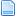Author Topic: How far can I push axis formulas?  (Read 3457 times)

0 Members and 1 Guest are viewing this topic.

####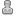abhi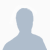•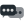24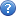##### How far can I push axis formulas?
« on: July 22, 2012, 07:46:09 AM »
Im wanting to attempt something of a large equation and in axis formulas and wondering if anyone has tried something like this.

this is what it looks like in code with variables, it would get a bit longer (lol) without the variables due to repetition. Am I barking out the wrong tree?

for iy in 1 to 10 do
(
for ix in 1 to 10 do
(
y=iy
x=ix
Ymax = 10
Xmax = 10
Tx = x/Xmax
Ty = y/Ymax
X_1a= 0.0
X_1b= 5.0
X_1c= 0.0
X_3a= 20.0
X_3b= 500.0
X_3c= 10.0
X_5a= 5.0
X_5b= 200
X_5c= 10.0
X_2a= X_3a*0.1+X_1a
X_4a= X_3a*0.1+X_5a
X_2b= X_3b*0.1+X_1b
X_4b= X_3b*0.1+X_5b
X_2c= X_3c*0.1+X_1c
X_4c= X_3c*0.1+X_5c
Y_1= (1.0-Tx)^4.0*X_1a+4.0*(1.0-Tx)^3*Tx*X_2a+4*(1.0-Tx)^2*Tx^2*X_3a+4.0*(1.0-Tx)*Tx^3*X_4a+Tx^4*X_5a
Y_3 =(1.0-Tx)^4.0*X_1b+4.0*(1.0-Tx)^3*Tx*X_2b+4*(1.0-Tx)^2*Tx^2*X_3b+4.0*(1.0-Tx)*Tx^3*X_4b+Tx^4*X_5b
Y_5 =(1.0-Tx)^4.0*X_1c+4.0*(1.0-Tx)^3*Tx*X_2c+4*(1.0-Tx)^2*Tx^2*X_3c+4.0*(1.0-Tx)*Tx^3*X_4c+Tx^4*X_5c
Y_2 = Y_3*0.01+Y_1
Y_4 = Y_3*0.01+Y_5

zPos =(1.0-Ty)^4.0*Y_1+4.0*(1.0-Ty)^3*Ty*Y_2+4*(1.0-Ty)^2*Ty^2*Y_3+4.0*(1.0-Ty)*Ty^3*Y_4+Ty^4*Y_5
Point pos:[ix*10,iy*10,zPos] size:1
)
)
this code makes an array of points using a modified bezier equation in 3DSmax. Im also thinking of ways to shorten it but keep on wishing for arbitrary variables.

Is is possible to do this with VB, I've not tried my hand with that so I don't really know how difficult it would be.

####BR549•6,952##### Re: How far can I push axis formulas?
« Reply #1 on: July 22, 2012, 10:25:59 AM »

(;-) TP

####abhi•24##### Re: How far can I push axis formulas?
« Reply #2 on: July 22, 2012, 10:44:44 AM »
its good exersise####BR549•6,952##### Re: How far can I push axis formulas?
« Reply #3 on: July 22, 2012, 12:00:02 PM »
Have you looked at the axis formula section in MACH3??   How do you plan to get all that to work in a simple linear equation that fits on 1 line per axis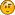Mach3 has 2 simple sections for axis corrections one being the screw mapping and the other is a simple alignment section the axis formulas.

A good exercise would be to fix the machine then get busy making chips.

(;-) TP

####Ya-Nvr-No•992##### Re: How far can I push axis formulas?
« Reply #4 on: July 22, 2012, 07:34:28 PM »
I used this program I developed to cut a 90 degree radius on a profile cut, but have to admit I have to single block the VB Code to get it to work. Some reason it wont run in auto. But it did get the job done, Made a oval table top for a walnut pedestal I refinished over 10 years ago.

But I have to agree: "FIX YOUR MACHINE." Your spending more time with creating bandage's then it would take to fix it.

Sub main()
Dim z,x,arcdist As Double
Dim steps As Integer

xpos=-12.2116 'outside dimension in x
zpos=-.45 'face start depth
xpos3=0
zpos3=0
z=(Cos(8.182/180*3.1416)*arcdist)
x=(Sin(8.182/180*3.1416)*arcdist)
'sleep 1000
steps=26 ' number of profile cuts
ang=90/steps
ang2=0
For p=1 To steps
ang2=ang2+ang
x=(Cos(ang2/180*3.1416)*arcdist)
z=(Sin(ang2/180*3.1416)*arcdist)
xpos2=xpos+(-x)
zpos2=-arcdist+zpos+z
xpos3=(xpos2+arcdist)/xpos
zpos3=zpos2/zpos
'this is where I alter the scaling to create the arch position
setoemdro(59,xpos3)
setoemdro(60,xpos3)
setoemdro(61,zpos3)

runfile()
Sleep(1000)
While IsMoving()
Sleep(1000)
Wend
Next
End Sub

main

####abhi•24##### Re: How far can I push axis formulas?
« Reply #5 on: July 23, 2012, 09:00:29 AM »
lol Im sensing a trend.
I plan to fix, its a priority.
Hey thanks for sharing the code, it looks like mach3 has a fair few hooks for vb just at a glance. Ill give it a spin since it doesn't look to different from what Im used to working with.

####Ya-Nvr-No•992##### Re: How far can I push axis formulas?
« Reply #6 on: July 23, 2012, 04:15:55 PM »
It was just to show you what is possible, but it has its limitations. Hopefully mach4 with something other than VB will be a fix all.

####abhi•24##### Re: How far can I push axis formulas?
« Reply #7 on: July 23, 2012, 07:29:44 PM »
oh okay, i started looking at specifics with cypress etc. I will no doubt look through more code so as to stand on the shoulders of giants so to speak.

originally my formula was much shorter but after doing propper measurements and plotting them on a graph i discovered that the deflection curve shape was a "bell" rather than a simple curve but now I'm thinking to accept the +/- 0.01 mm that I'm chasing through the higher level equation and take my time learning the deeper mysteries of mach3.

oh.... is mach4 slated for release? as best i could tell its not quite a ship on the horrizon.